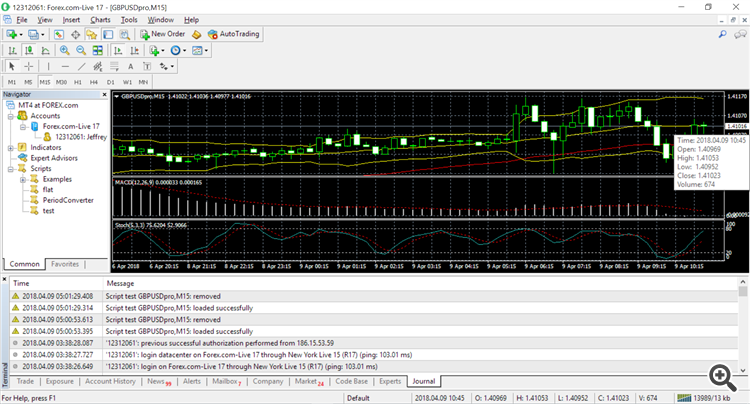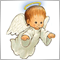# open high low close not matching charts608

When I run the script below my values on the chart do not match the output.  I am using NULL for the symbol name parameter of the CopyTime function.  According to the documentation I thought that meant the current chart's currency pair would be used but they do not match.  I have included the script and last line of the output file along with a screen shot of the only chart I have open showing the values of the last candle.

2018.04.09 10:55|1.27910000|1.28010000|1.27903000|1.27918000```#property copyright   "2005-2014, MetaQuotes Software Corp."
#property description "Moving Averages Convergence/Divergence"
#property strict

void OnStart()
{

double   ma1O[];
double   ma1H[];
double   ma1L[];
double   ma1C[];
datetime date_buff[];
string   line;

int copied=CopyTime(NULL,PERIOD_M5,D'2018.02.01 01:01:01',D'2019.01.01 01:01:01',date_buff);
ArrayResize(ma1O,copied);
ArrayResize(ma1H,copied);
ArrayResize(ma1L,copied);
ArrayResize(ma1C,copied);
for(int i=0;i<copied;i++)
{
ma1O[i]   =Open[i];
ma1H[i]   =High[i];
ma1L[i]   =Low[i];
ma1C[i]   =Close[i];
line=TimeToString(date_buff[i])
+"|"+DoubleToString(ma1O[i])
+"|"+DoubleToString(ma1H[i])
+"|"+DoubleToString(ma1L[i])
+"|"+DoubleToString(ma1C[i]);
FileWrite(file_handle,line);
}
FileClose(file_handle);
}
```3024

jshumaker:

When I run the script below my values on the chart do not match the output.  I am using NULL for the symbol name parameter of the CopyTime function.  According to the documentation I thought that meant the current chart's currency pair would be used but they do not match.  I have included the script and last line of the output file along with a screen shot of the only chart I have open showing the values of the last candle.

```void OnStart()
{

double   ma1O[];
double   ma1H[];
double   ma1L[];
double   ma1C[];
datetime date_buff[];
string   line;

int copied=CopyTime(_Symbol,_Period,D'2018.02.01 01:01:01',D'2019.01.01 01:01:01',date_buff);
copied=CopyOpen(_Symbol,_Period,D'2018.02.01 01:01:01',D'2019.01.01 01:01:01',ma1O);
copied=CopyHigh(_Symbol,_Period,D'2018.02.01 01:01:01',D'2019.01.01 01:01:01',ma1H);
copied=CopyLow(_Symbol,_Period,D'2018.02.01 01:01:01',D'2019.01.01 01:01:01',ma1L);
copied=CopyClose(_Symbol,_Period,D'2018.02.01 01:01:01',D'2019.01.01 01:01:01',ma1C);
ArraySetAsSeries(date_buff,true);
ArraySetAsSeries(ma1O,true);
ArraySetAsSeries(ma1H,true);
ArraySetAsSeries(ma1O,true);
ArraySetAsSeries(ma1C,true);
for(int i=0;i<copied;i++)
{
line=TimeToString(date_buff[i])
+"|"+DoubleToString(ma1O[i])
+"|"+DoubleToString(ma1H[i])
+"|"+DoubleToString(ma1L[i])
+"|"+DoubleToString(ma1C[i]);
FileWrite(file_handle,line);
}
FileClose(file_handle);
}```